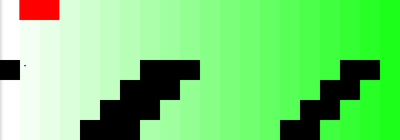# NanoBomber - 694B

A Canyon bomber in <1K! (694B exactly) Press space to start and score as many points as possible in one minute!Hewitt Squared Labs
(hewitt2labs)
A game inspired by a couple of snake games being made as byte compact as possible. Press space to drop a bomb, the squares to the right are worth more points. (the ones that turn black anyways)

### Code

Warning! code is currently broken! see below for details
```from pygame import *
D=display;L=D.set_mode((640,224)).fill;P=B=F=C=E=0;M=32;H=D.set_caption;
def I():
for e in event.get():
if e.type==12:z
if e.type==2:
if e.key==27:z
if e.key==32:return 1
while 1:
time.Clock().tick(10)
if E>0:
P-=32
if P<0:P=639
if F:B+=32
else:F=I()
if B>223:F=0;B=0
if B>64:C+=T[B/M][P/M];T[B/M][P/M]=0
E-=0.1;H("Score:%s Time:%s"%(C,E))
else:
H("Game Over Score:%s (space to start)"%(C));F=I()
if F:E=60;P=F=B=C=0;T=[[[x+1,x,y]for x in range(20)]for y in range(7)]
L(0)
for t in T:
for s in t:
g,x,y=s
if g:
j=255-x*12;L((j,255,j),((x*M,y*M),(M,M)))
L(255,((P+8,B+8),(2,2)));L(16711680,((P,0),(M*2,M)));D.flip()```
Seriously considering a easier to read dev version!

### Notes

• Byte count is using LF (linefeed) endings NOT CRLF(cairrage-return/linefeed) since gedit won't use CRLF.
• There is probably many things that could be done to make it shorter(much shorter) but it's working! (sort of :))

### Agenda

(Numbered in order of pseudo-importance)
1. Make "plane" fly all the time and only activate "bombs" when timer is going down.(It might make the code smaller)
2. Make the top two rows of "blocks" black. They're not because
`T[:2][:]=[0,0,0]`
won't make the first 40 sub-sub lists of T = [0,0,0]! (thinking about it, it should actually be:
`T[:2][:]=0`
to change the color...)
3. Move "plane" in pixel increments instead of 32p blocks
4. Make "bomb" bigger. (Hard to see right now)

### Changes